+
Solving Problems involving Percents
Solving Linear Equations and Inequalities
0
of 0 possible points

# Solving Problems involving Percents

Author: Sophia Tutorial
##### Description:

Calculate the original price of an item that had either a sales tax or discount applied.

(more)

Sophia’s self-paced online courses are a great way to save time and money as you earn credits eligible for transfer to many different colleges and universities.*

No credit card required

37 Sophia partners guarantee credit transfer.

299 Institutions have accepted or given pre-approval for credit transfer.

* The American Council on Education's College Credit Recommendation Service (ACE Credit®) has evaluated and recommended college credit for 33 of Sophia’s online courses. Many different colleges and universities consider ACE CREDIT recommendations in determining the applicability to their course and degree programs.

Tutorial
what's covered
1. Percent Tax
2. Percent Discount

# 1. Percent Tax

Many items that we purchase every day have tax applied to them. Tax represents an increase in the price we must pay for these items, so it's intuitive to think of the original price to be multiplied by a number larger than 1. Tax is also most often a percentage rate, such as 7%. When adding 7% of a quantity to itself, you are really doing two things: first, you are calculating 7% of that item, and then adding it to 100% of the initial amount. For this reason, we use the factor (1+r) to calculate the price of an item with tax included. It is important to note that r is the tax rate expressed as a decimal, and the 1 represents 100% of the original price.

To calculate the price of an item with tax included, multiply the original price of the item by (1+r), where r is the percent tax expressed as a decimal.

formula
Tax Formula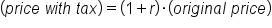Let's apply this concept to a few basic examples.

A pair of pants costs \$18. There is a 6% tax applied on to the cost of the pants. How much is due at checkout for the pants?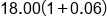6% is expressed as 0.06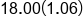Sum 1 and 0.06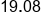Multiply 18 by 1.06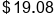Our Solution

Percent tax problems can get complicated. Consider the next scenario.

You manage an art store, and are preparing an order for canvas paper from your distributor. You purchase 40 boxes of canvas paper, and the total comes to \$998.20, which includes an 8.5% tax. What is the cost of each box of canvas paper before taxes?

Here, the figure of \$998.20 already includes the 8.5% tax, so as we solve our problem, we have to keep in mind that some dollar amount has increased by 8.5% to 998.2. That dollar amount represents the cost of 40 boxes of canvas paper before tax was applied. A final step will be to divide the cost by the number of boxes to represent the cost of each box before taxes. Our solution is worked out below: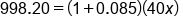8.5% is expressed as 0.085, x=cost of boxes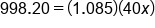Sum 1 and 0.085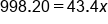Multiply 1.085 by 40x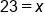Divide by 43.4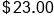Our Solution

# 2. Percent Discount

Just as percent tax represents an increase in cost, percent discount represents a decrease in cost. Recall that we multiplied the cost of an item by (1+r) to represent tax being applied. Similarly, we can multiply the cost of an item by (1-r), where r is the percent discount.

formula
Discount Formula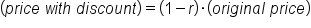One way to think about (1–r) is that we are retaining a certain percentage of the cost after a discount, and that percentage is 100% of the cost minus the percent discount. For example, if the percent discount is 30%, we must still pay for 70% of the item.

Let's take a look at a basic percent discount problem, and then we will tackle a more complicated one:

Last week, a patio umbrella was priced at \$149.99. This week, the item is on sale with a 20% discount. How much does the patio umbrella cost this week?

To solve this problem, first we must represent 20% as a decimal, and subtract it from 1. This is the (1–r) factor that will be multiplied by the original price to give us the price after the discount. The solution is worked out below: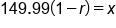x=price after discount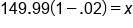20% discount =(1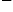.02)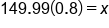Subtract 0.2 from 1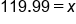Multiply 149.99 by 0.8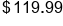Our Solution

Our final example is more complicated, because it includes both sales tax and a percent discount. Consider the following:

You are placing an online order to purchase 8 novels for a book club meeting. The total amount due is \$89.88, which includes a 25% discount on the books, and a 7% sales tax. What is the price of each book, before any discounts or taxes are applied?

In this situation, we are dealing with two rates. The first rate is the percent discount of 25%. As a factor in our equation, we will use (1–0.25) to represent the percent discount. The second rate is the sales tax of 7%. The factor for this will be (1+0.07). The solution to our problem is worked out below: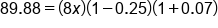x=price of each book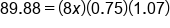Simplify in parentheses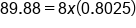Multiply 0.75 by 1.07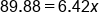Multiply 8x by 0.8025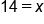Divide by 6.42

summary
Percentages can be used for solving everyday problems such as those involving tax or discounts. Percent tax represents an increase in cost, percent discount represents a decrease in cost. When calculating the percentages, you have to convert the percentage to decimal form by dividing by 100.

Rating